﻿ SQL Square Brackets - TutorialsTrend
This Angular tutorial helps you get started with Angular quickly and effectively through many practical examples.
`

# SQL-Square Brackets

In this tutorial, We will learn how to use Square Brackets with table name, column name and keyword in SQL Server.

#### SQL Server Square Brackets

Square brackets are one of the worst things that Microsoft has put into the SQL server. When you execute a query in SQL, you often have already noticed a common error when creating a table, and the table name contains a space like a Employee table. It will show an error message like "Incorrect syntax near the table." It's because of the square brackets.

The solution is very simple, put the table name in square brackets. You might encounter columns named keywords. In that case, also you need to use square brackets for that particular column name. So let's look at a practical example of where to use square brackets in SQL Server.

Square Brackets can be used in a variety of situations.

#### Problem 1. Creating a Table Name with Space in SQL Server

When you create a table with the table name containing a space like in the Employee table, it will show an error message like "Incorrect syntax near the table." The following creates a table script.

``````CREATE TABLE Employee table
(
[emp_id] [int] NULL,
[emp_name] [varchar](20) NULL,
[Email] [varchar](100) NULL,
[Remarks] [varchar](50) NULL
)``````

Now press F5 to execute it. It will show an error message.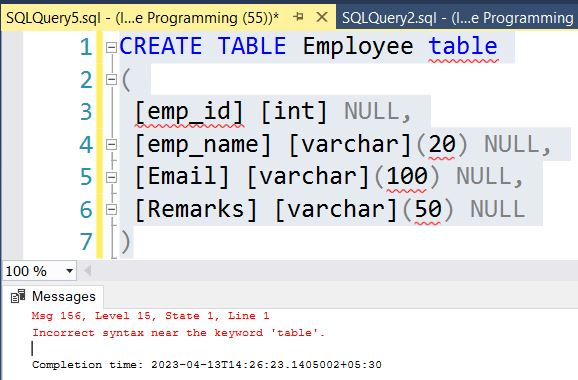The above error is because of square brackets. The solution is very simple, put the table name in square brackets.

``````CREATE TABLE [Employee table]
(
[emp_id] [int] NULL,
[emp_name] [varchar](20) NULL,
[Email] [varchar](100) NULL,
[Remarks] [varchar](50) NULL
)``````

NNow press F5 to execute it.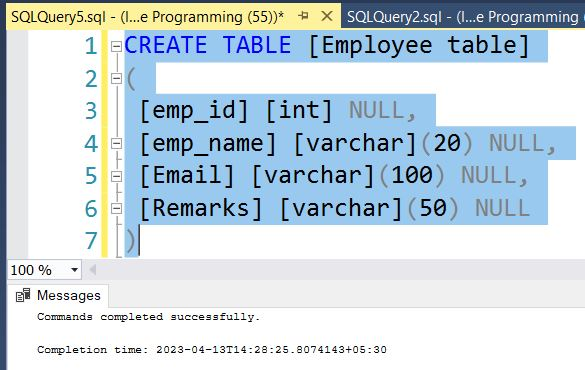#### Problem 2. Using Columns Name with Space SQL Keywords

When you create a table with the column name containing a space like in the Employeetable, it will show an error message like "Incorrect syntax near the table." The following creates a table script.

``````CREATE TABLE [Employee table]
(
[emp_id] [int] NULL,
[emp_name] [varchar](20) NULL,
[Email] [varchar](100) NULL,
[Remarks] [varchar](50) NULL,
Emp desig [varchar](20) NULL
)``````

Now Press F5 to execute it. It will show an error message.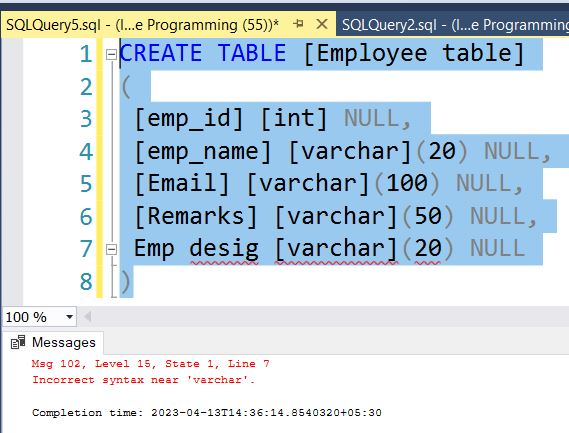The above error is because of square brackets. The solution is very simple, put the column name in square brackets.

``````CREATE TABLE [Employee table]
(
[emp_id] [int] NULL,
[emp_name] [varchar](20) NULL,
[Email] [varchar](100) NULL,
[Remarks] [varchar](50) NULL,
[Emp desig] [varchar](20) NULL
)``````

Now Press F5 to execute it.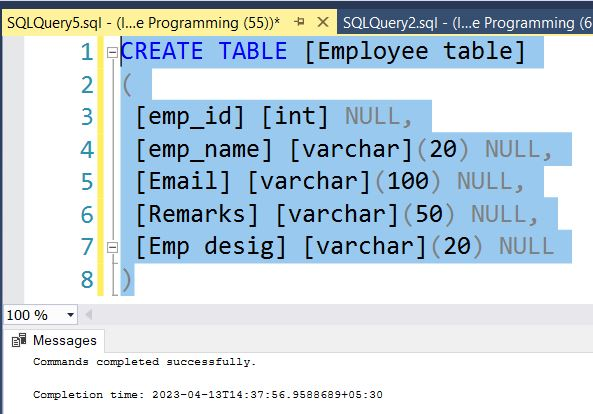#### Problem 3. Using Columns Name as SQL Keywords

You define a column name that is also a keyword. The following creates a table with a column named FROM, a keyword script. It will show an error message like "Incorrect syntax near keyword".

``````CREATE TABLE [Employee table]
(
[emp_id] [int] NULL,
[emp_name] [varchar](20) NULL,
[Email] [varchar](100) NULL,
[Remarks] [varchar](50) NULL,
From [varchar](20) NULL
)``````

Now Press F5 to execute it. It will show an error message.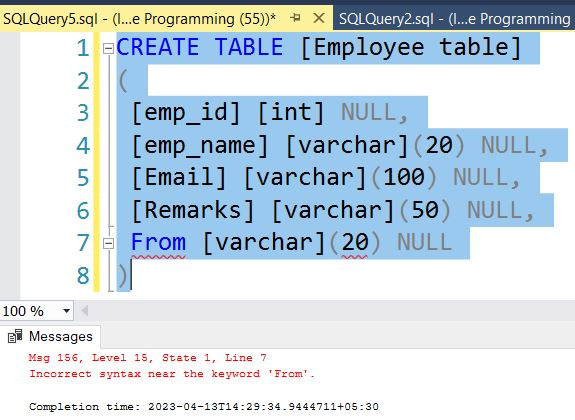The above error is because of square brackets. The solution is very simple, put the table name in square brackets.

``````CREATE TABLE [Employee table]
(
[emp_id] [int] NULL,
[emp_name] [varchar](20) NULL,
[Email] [varchar](100) NULL,
[Remarks] [varchar](50) NULL,
[From] [varchar](20) NULL
)``````

Now Press F5 to execute it.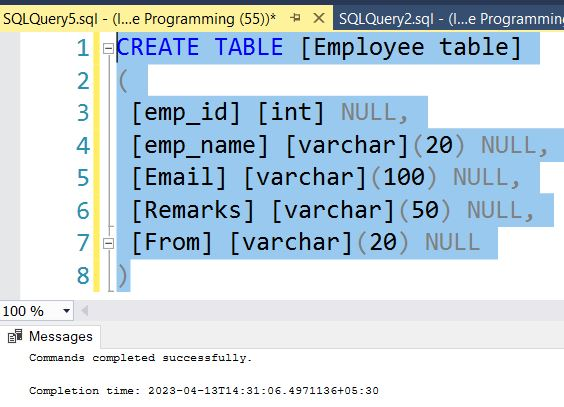Next